## Monday, 23 July 2012

### CBSE Class 11 - Physics - CH2 - Error Analysis

Error Analysis

Q1: Define error.

Answer: The exact  measurement of a physical quaintly not possible. This is known as Heisenberg uncertainly principle. Uncertainty in the measurements is called error.

Numerically, the deviation of measured value from the actual value is called error.  If X is the quantity measured, then ΔX is called error in X. i.e. Error = Actual value - Measured value.

Q2: What are different sources of errors?

Answer: An error can be of following types:
(a) systematic errors
(b) random errors.
(c) least count errors
(d) constant errors
(e) Errors due to external factors.

(a) Systematic error is the deviation caused due a single known source is called a systematic error. Following are the sources of systematic errors:

• Instrumental errors: Imperfect design or calibration and/or Zero error.
• Imperfection in experimental technique or procedure
• Personal errors or Gross errors due to individual’s bias, lack of proper setting of the apparatus or individual’s carelessness in taking observations without observing proper precautions,

(b) Random Error: Error due to unknown reasons and that occurs while taking measurements.

(c) The least count error is the error associated with the resolution of the instrument.

(d) Constant Errors. Error in magnitude caused due to problems in calibration of scales. This error repeats itself in all measurements. Least Count error is also a type of constant error.

(e) External factors like air, temperature, pressure etc. can cause errors while taking measurements.

Q3: How can we minimize errors?

1. Using instruments of higher precision, improving experimental techniques, etc., we can
reduce the least count error
2. Repeating the observations several times and taking the arithmetic mean of all the observations, the mean value would be very close to the true value of the measured quantity.
3. Gross errors can be minimized only if the observer is very careful in his observations and sincere in his approach.
Q4: What are the different ways of expressing an error?

Answer: different ways to express errors are:
• Absolute Error
• Relative Error
• Percentage Error

Q5: What is Absolute Error?

Answer:  The absolute error of measurement is the magnitude of the difference between the value of the quantity and the individual measurement value. Because we are not sure about the correct value of the quantity, correct or true value of quantity is taken as Arithmetic mean (Amean) of read values.

If there are 'n' number of readings (A1, A2, A3 ... An), corresponding absolute errors can be represented as:
ΔA1 = Amean - A1
ΔA2 = Amean - A2
ΔA3 = Amean - A3
...
ΔAn = Amean - An

Final Absolute error is arithmetic mean of magnitudes of absolute errors i.e.
ΔAmean= ( |ΔA1|+ |ΔA2| + |ΔA3|+ ... + |ΔAn|) / n

Q6: Define accuracy.

Answer: The accuracy of an instrument is a measure of how close the output reading of the instrument is to the correct value.

Q7: What is meant by precision? Can we say an instrument of high precision is accurate?

Answer: Precision describes an instrument’s degree of freedom from random errors. If a large number of readings are taken of the same quantity by a high precision instrument, then the spread of readings will be very small. It is not necessary an instrument of high precision is accurate.

Q8: Any measurement of physical quantity p will likely to have value
(a) Always equal to pmean - Δpmean
(b) Always equal to pmean + Δpmean
(c) Greater than  pmean + Δpmean
(d) Lies between  pmean - Δpmean ≤  p  ≤  pmean + Δpmean

Answer:  (d) Lies between  pmean - Δpmean ≤  p  ≤  pmean + Δpmean

Q9: What is relative error or percentage error?

Answer: The relative error is the ratio of the mean absolute error Δamean to the mean value amean of the quantity measured.
i.e. Relative error = Δamean/ amean

When the relative error is expressed in per cent, it is called the percentage error (δa).
⇒  δa = (Δamean/ amean) × 100%

Q10: A resistor has a marking as “470 Ω, 10%”? What will be the true value of resistor?

Answer: 470 Ω, 10% means, the resistor has percentage error of 10%. it means the true value lies withing 470 ± 10% i.e. 470 ± 47. (10% of 470 is 47)
∴ true value lies between 423Ω to 517 Ω.

Q11: The refractive index (μ) of water is found to have the values 1.29, 1.33, 1.34, 1.35, 1.32, 1.36, 1.30 and 1.33. Calculate the mean value, absolute error, the relative error and the percentage error.

Answer: Mean refractive index is average of eight values i.e.
μmean = (1.29 + 1.33 + 1.34 + 1.35 + 1.32 + 1.36 + 1.30 + 1.33) ÷ 8
μmean =10.62 ÷ 8 = 1.3275 ≈ 1.33

Absolute error are:
Δμ1 = μmean - μ1  = 1.33 - 1.29 = 0.04
Δμ2 = μmean - μ2  = 1.33 - 1.33 = 0.00
Δμ3 = μmean - μ3  = 1.33 - 1.34 = -0.01
Δμ4 = μmean - μ4  = 1.33 - 1.35 = -0.02
Δμ5 = μmean - μ5  = 1.33 - 1.32 = 0.01
Δμ6 = μmean - μ6  =  1.33 - 1.36 = -0.03
Δμ7 = μmean - μ7  = 1.33 - 1.30 = 0.03
Δμ8 = μmean - μ8  =  1.33 - 1.33 = 0.00

Mean Absolute error  Δμmean
= (|Δμ1 | + |Δμ2 | + |Δμ3 | + | Δμ4 | + | Δμ5 | + | Δμ6 | + |Δμ7 | + |Δμ8 |) ÷ 8
= ( 0.04 + 0.00 + 0.01 + 0.02 + 0.01 + 0.03 + 0.03 + 0.00) ÷ 8
= 0.14 ÷ 8 = 0.0175 ≈  0.02

Relative error (δμ) Δμmean/ μmean = 0.02 ÷ 1.33 = ± 0.015 0.02

Percentage error =  (Δμmean/ μmean) × 100% = ± 0.015 × 100 = ± 1.5

Q12: Static analysis is used to handle
(a) Systematic errors
(b) Random errors
(c) Constant errors
(d) Least Count errors

Answer: (b) Random errors

Q13: What is Parallax error?

Answer:  Parallax error is a type of gross error which gets introduced by reading scales from the wrong angle i.e. any angle other than at right angle. Usually some instruments have mirrors help to avoid parallax error while taking readings.

Q14: How errors are propagated or combined?

Answer: While applying mathematical operations (e.g. addition, subtraction, multiplication and division) on physical quantities, errors are combined.

If A and B are two physical quantities represented as A ± ΔA and B ± ΔB, then
(a) In addition, i.e. Z = A + B, absolute error  ΔZ = ΔA + ΔB.

(b) In difference, Z = A - B, absolute error  ΔZ = ΔA + ΔB.

(c) In product, Z = AB, then absolute error  ΔZ/Z = ΔA/A + ΔB/B.

(d) In division, Z = A/B,  ΔZ/Z = ΔA/A + ΔB/B.

(e) In power, Z = Ax.By/Cz, then  ΔZ/Z = xΔA/A + yΔB/B ± zΔC/C

Q15: A current of 3.5 ± 0·5 ampere flows through metallic conductor.  And a potential difference of 21 ± 1 volt is applied. Find the Resistance of the wire.

Answer:  Given V = 21± 1volts, ΔV = 1, I = 3.5 ± 0·5 A and ΔI = 0.5A
Resistance R  = V / I = 21± 1 / 3.5 ± 0·5 = 6 ± ΔR
ΔR/R = error in measurement = ΔV/V + ΔI/I = 1/21 + 0.5/3.5 = 0.048 + 0.143 = 0.191 ≈ 0.19
⇒ ΔR = 0.19 × R = 0.19 × 6 = 1.14

∴ effective resistance R = 6 ± 1.14 Ω

Q16: A rectangular board is measured with a scale having accuracy of 0.2cm. The length and breadth are measured as 35.4 cm and 18.4 cm respective. Find the relative error and percentage error of the area calculated.

Answer: Given length (l) = 35.4 cm, Δl = 0.2cm
Width (w) = 18.4cm and Δw = 0.2cm

Area (A) = l × w = 35.4 × 18.4 = 651.36 cm2

Relative error in Area (δA) = ΔA/A = Δl/l + Δw/w =0.2/35.4 + 0.2/18.4 = 0.006 + 0.011 = 0.017
Percentage error = ΔA/A × 100 = 0.017 × 100 = 1.7%

(In progress...)1.1.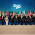This comment has been removed by the author.

2.Easy to understand....

2.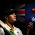3.4.5.6.This comment has been removed by the author.

7.veryyyyy veryyyy niceeeeeeeee

8.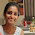9.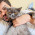10.Thanx a lot...

11.12.clear concept

13.Helped me a lot

14.15.16.Kuch samaj me nahi aya. Bekar answer kikha hai

17.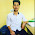18.